# HSSlive: Plus One & Plus Two Notes & Solutions for Kerala State Board

## AP Board Class 8 Maths Chapter 6 Square Roots and Cube Roots InText Questions Textbook Solutions PDF: Download Andhra Pradesh Board STD 8th Maths Chapter 6 Square Roots and Cube Roots InText Questions Book AnswersAP Board Class 8 Maths Chapter 6 Square Roots and Cube Roots InText Questions Textbook Solutions PDF: Download Andhra Pradesh Board STD 8th Maths Chapter 6 Square Roots and Cube Roots InText Questions Book Answers

## Andhra Pradesh State Board Class 8th Maths Chapter 6 Square Roots and Cube Roots InText Questions Books Solutions

 Board AP Board Materials Textbook Solutions/Guide Format DOC/PDF Class 8th Subject Maths Chapters Maths Chapter 6 Square Roots and Cube Roots InText Questions Provider Hsslive

## How to download Andhra Pradesh Board Class 8th Maths Chapter 6 Square Roots and Cube Roots InText Questions Textbook Solutions Answers PDF Online?

2. Click on the Andhra Pradesh Board Class 8th Maths Chapter 6 Square Roots and Cube Roots InText Questions Answers.
3. Look for your Andhra Pradesh Board STD 8th Maths Chapter 6 Square Roots and Cube Roots InText Questions Textbooks PDF.
4. Now download or read the Andhra Pradesh Board Class 8th Maths Chapter 6 Square Roots and Cube Roots InText Questions Textbook Solutions for PDF Free.

## AP Board Class 8th Maths Chapter 6 Square Roots and Cube Roots InText Questions Textbooks Solutions with Answer PDF Download

Find below the list of all AP Board Class 8th Maths Chapter 6 Square Roots and Cube Roots InText Questions Textbook Solutions for PDF’s for you to download and prepare for the upcoming exams:

### 8th Class Maths 6th Lesson Square Roots and Cube Roots InText Questions and Answers

Do this

Question 1.
Find the perfect squares between (i) 100 and 150 (ii) 150 and 200 [Page No. 124]
i) The perfect squares between 100 and 150 are = 121, 144
ii) Perfect squares between 150 and 200 = 169, 196

Question 2.
Is 56 a perfect square? Give reasons. [Page No. 124]
Product of primes of 56 = 8 × 7 = (2 × 2) × 2 × 7
56 is not a perfect square. Since it can’t be written as product of two same numbers.

Question 3.
How many non perfect square numbers are there between 92 and 102? [Page No. 128]
No. of non perfect square numbers between 92 and 102 are
= 2 × base of first number = 2 × 9 = 18
They are 82, 83, ……. 99.

Question 4.
How many non perfect square numbers are there between 152 and 162? [Page No. 128]
No. of non perfect square numbers between 15 and 16 are = 2 × base of first number = 2 × 15 = 30
They are 226, 227, ……. 255,

Question 5.
Check whether the following numbers form pythagorean triplet. [Page No. 129]
(i) 2, 3, 4
(ii) 6, 8, 10
(iii) 9, 10, 11
(iv) 8,15, 17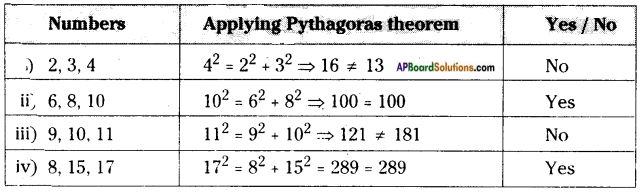Question 6.
Take a pythagorean triplet. Write their multiples. Check whether these multiples form a pythagorean triplet. [Page No. 129]
3, 4, 5 are pythagorean triplets.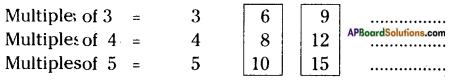From 6,8,10
⇒ 102 = 82 + 62
⇒ 100 = 64 + 36
⇒ 100 = 100 (T)
From 9, 12, 5
⇒ 152 = 92 + 122
⇒ 225 = 81 + 144
⇒ 225 = 225 (T)
∴ The multiples of pythagorean triplets are also pythagorean triplets.

Question 7.
By subtraction of successive odd numbers And whether the following numbers are perfect squares or not. [Page No. 131]
(i) 55 (ii) 90 (iii) 121
(i) √55
Step 1 → 55 – 1 = 54 (1st odd number be subtracted)
Step 2 → 54 – 3 = 51 (2nd odd number be subtracted)
Step 3 → 51 – 5 = 46 (3rd odd number be subtracted)
Step 4 → 46 – 7 = 39 (4th odd number be subtracted)
Step 5 → 39 – 9 = 30 (5th odd number be subtracted)
Step 6 → 30 – 11 = 19 (6th odd number be subtracted)
Step 7 → 19 – 13 = 6 (7th odd number be subtracted)
∴ 55 is not a perfect square number.
(∵ difference of consecutive odd numbers is not equal to ‘0’)

ii) √90
Step 1 → 90 – 1 =89 (1st odd number be subtracted)
Step 2 → 89 – 3 = 86 (2nd odd number be subtracted)
Step 3 → 86 – 5 = 81 (3rd odd number be subtracted)
Step 4 → 81 – 7 = 74 (4th odd number be subtracted)
Step 5 → 74 – 9 = 65 (5th odd number be subtracted)
Step 6 → 65 – 11 = 54 (6th odd number be subtracted)
Step 7 → 54 – 13 = 41 (7th odd number be subtracted)
Step 8 → 41 – 15 = 26 (8th odd number be subtracted)
Step 9 → 26 – 17 = 9 (9th odd number be subtracted)
∴ 90 is not a perfect square number.
(∵ difference of consecutive odd numbers is not equal to ‘0’)

iii) √121
Step 1 → 121 – 1 = 120 (1st odd number is subtracted)
Step 2 → 120 – 3 = 117 (2nd odd number is subtracted)
Step 3 → 117 – 5 = 112 (3rd odd number is subtracted)
Step 4 → 112 – 7 = 105 (4th odd number is subtracted)
Step 5 → 105 – 9 = 96 (5th odd number is subtracted)
Step 6 → 96 – 11 = 85 (6th odd number is subtracted)
Step 7 → 85 – 13 = 72 (7th odd number is subtracted)
Step 8 → 72 – 15 = 57 (8th odd number is subtracted)
Step 9 → 57 – 17 = 40 (9th odd number is subtracted)
Step 10 → 40 – 19 = 21 (10th odd number is subtracted)
Step 11 → 21 – 21 = 0 (11th odd number is subtracted)
∴ At the 11th step, the difference of consecutive odd numbers is ‘0’
121 is a perfect square number.
∴ √121 = 11×11‾‾‾‾‾‾‾√ = 11 (∵ It ends at 11th step)

Question 8.
Which of the following are perfect cubes? [Page No. 143]
(i) 243 (ii) 400 (iii) 500 (iv) 512 (v) 729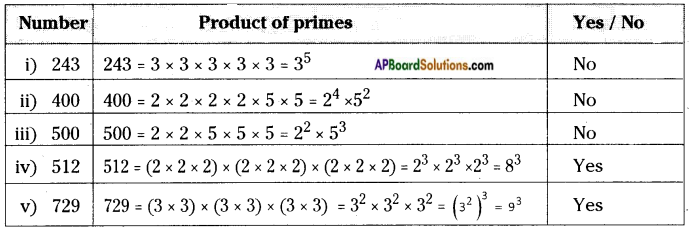∴ 512 and 729 are perfect cubes.

Try These

Question 1.
Guess and give reason which of the following numbers are perfect squares. Verify from the above table. (Refer table in Text Page no: 124) [Page No. 124]
(i) 84 (ii) 108 (iii) 271 (iv) 240 (v) 529
(i), (ii), (iii), (iv) are not perfect squares.
(v) 529 = 23 × 23
∴ 529 is a perfect square number.

Question 2.
Which of the following have one in its units place? [Page No. 125]
(i) 1262 (ii) 1792 (iii) 2812 (iv) 3632

 Number Square of units digit Units digit of a number i) 1262 (6)2 = 36 6 ii) 1792 (9)2 = 81 1 iii) 2812 (1)2 = 1 1 iv) 3632 (3)2 = 9 9

Question 3.
Which of the following have 6 in the units place?
(i) 1162 (ii) 2282 (iii) 3242 (iv) 3632  [Page No. 125]
i) 1162 ⇒ (6)2 = 36 units digit = 6
ii) 2282 ⇒ (8)2 = 64 units digit = 4
iii) 3242 ⇒ (4)2 = 16 units digit = 6
iv) 3632 ⇒ (3)2 = 9 units digit = 9
∴ Numbers which are having ‘6’ in its unit’s digit are: (i) 1162 (iii) 3242

Question 4.
Guess, how many digits are there in the squares of i) 72 ii) 103 iii) 1000 [Page No. 125]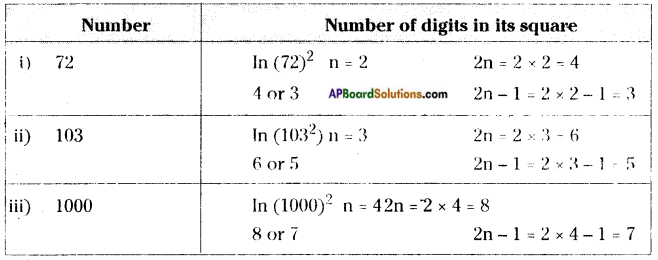Question 5.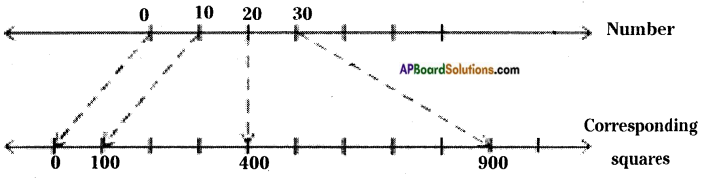27 lies between 20 and 30
272 lies between 202 and 302
Now find what would be 272 from the following perfect squares. [Page No. 125]
(i)329 (ii) 525 (iii) 529 (iv) 729
The value of (27)2 = 27 × 27 = 729

Question 6.
Rehan says there are 37 non square numbers between 92 and 112. Is he right? Give your reason. [Page No. 128 ]
No. of (integers) non perfect square numbers between 92 and 112
= 82, 83, ……. 100 …… 120 = 39
But 100 is a perfect square number.
∴ Required non perfect square numbers are = 39 – 1 = 38
∴ No, his assumption is wrong.

Question 7.
Is 81 a perfect cube? [Page No. 140]
81 = 3 × 3 × 3 × 3 = 34
No, 81 is not a perfect cube.
[∵ 81 can’t be written as product of 3 same numbers.]

Question 8.
Is 125 a perfect cube? [Page No.140]
125 = 5 × 5 × 5 = (5)3
Yes, 125 is a perfect cube.
[∵ It can be written as product of 3 same numbers]

Question 9.
Find the digit in units place of each of the following numbers. [Page No. 141]
(i) 753 (ii) 1233 (iii) 1573  (iv) 1983  (v) 2063

 Number Cube of a units digit Units digit i) 753 53= 125 5 ii) 1233 33 = 27 7 iii) 1573 73 = 343 3 iv) 1983 83 = 512 2 v) 2063 63 = 216 6

Think, Discuss and Write

Question 1.
Vaishnavi claims that the square of even numbers are even and that of odd are odd. Do you agree with her? Justify. [Page No. 125]
The square of an even number is an even
∵ The product of two even numbers is always an even.
Ex: (4)2 = 4 × 4 = 16 is ah even.
The square of an odd number is an odd.
∵ The product of two odd numbers is an odd number.
Ex: 112 = 11 × 11 = 121 is an odd.

Question 2.
Observe and complete the table: [Page No. 125]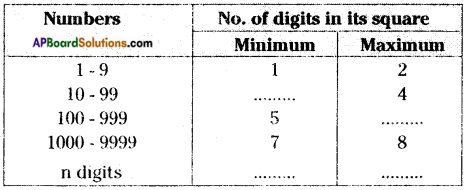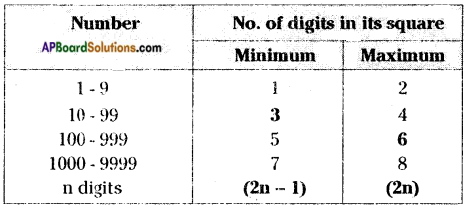Question 3.
How many perfect cube numbers are present between 1 and 100,1 and 500,1 and 1000? [Page No. 140]
Perfect cube numbers between 1 and 100 = 8, 27, 64
Perfect cube numbers between 1 and 500 = 8, 27, 64, 125, 216, 343
Perfect cube numbers between 1 and 1000 = 8, 27, 64, 125, 216, 343, 512, 729

Question 4.
How many perfect cubes are there between 500 and 1000? [Page No. 140]
Perfect cubes between 500 and 1000 = 512 and 729

## Andhra Pradesh Board Class 8th Maths Chapter 6 Square Roots and Cube Roots InText Questions Textbooks for Exam Preparations

Andhra Pradesh Board Class 8th Maths Chapter 6 Square Roots and Cube Roots InText Questions Textbook Solutions can be of great help in your Andhra Pradesh Board Class 8th Maths Chapter 6 Square Roots and Cube Roots InText Questions exam preparation. The AP Board STD 8th Maths Chapter 6 Square Roots and Cube Roots InText Questions Textbooks study material, used with the English medium textbooks, can help you complete the entire Class 8th Maths Chapter 6 Square Roots and Cube Roots InText Questions Books State Board syllabus with maximum efficiency.

## FAQs Regarding Andhra Pradesh Board Class 8th Maths Chapter 6 Square Roots and Cube Roots InText Questions Textbook Solutions

#### Can we get a Andhra Pradesh State Board Book PDF for all Classes?

Yes you can get Andhra Pradesh Board Text Book PDF for all classes using the links provided in the above article.

## Important Terms

Andhra Pradesh Board Class 8th Maths Chapter 6 Square Roots and Cube Roots InText Questions, AP Board Class 8th Maths Chapter 6 Square Roots and Cube Roots InText Questions Textbooks, Andhra Pradesh State Board Class 8th Maths Chapter 6 Square Roots and Cube Roots InText Questions, Andhra Pradesh State Board Class 8th Maths Chapter 6 Square Roots and Cube Roots InText Questions Textbook solutions, AP Board Class 8th Maths Chapter 6 Square Roots and Cube Roots InText Questions Textbooks Solutions, Andhra Pradesh Board STD 8th Maths Chapter 6 Square Roots and Cube Roots InText Questions, AP Board STD 8th Maths Chapter 6 Square Roots and Cube Roots InText Questions Textbooks, Andhra Pradesh State Board STD 8th Maths Chapter 6 Square Roots and Cube Roots InText Questions, Andhra Pradesh State Board STD 8th Maths Chapter 6 Square Roots and Cube Roots InText Questions Textbook solutions, AP Board STD 8th Maths Chapter 6 Square Roots and Cube Roots InText Questions Textbooks Solutions,
Share: